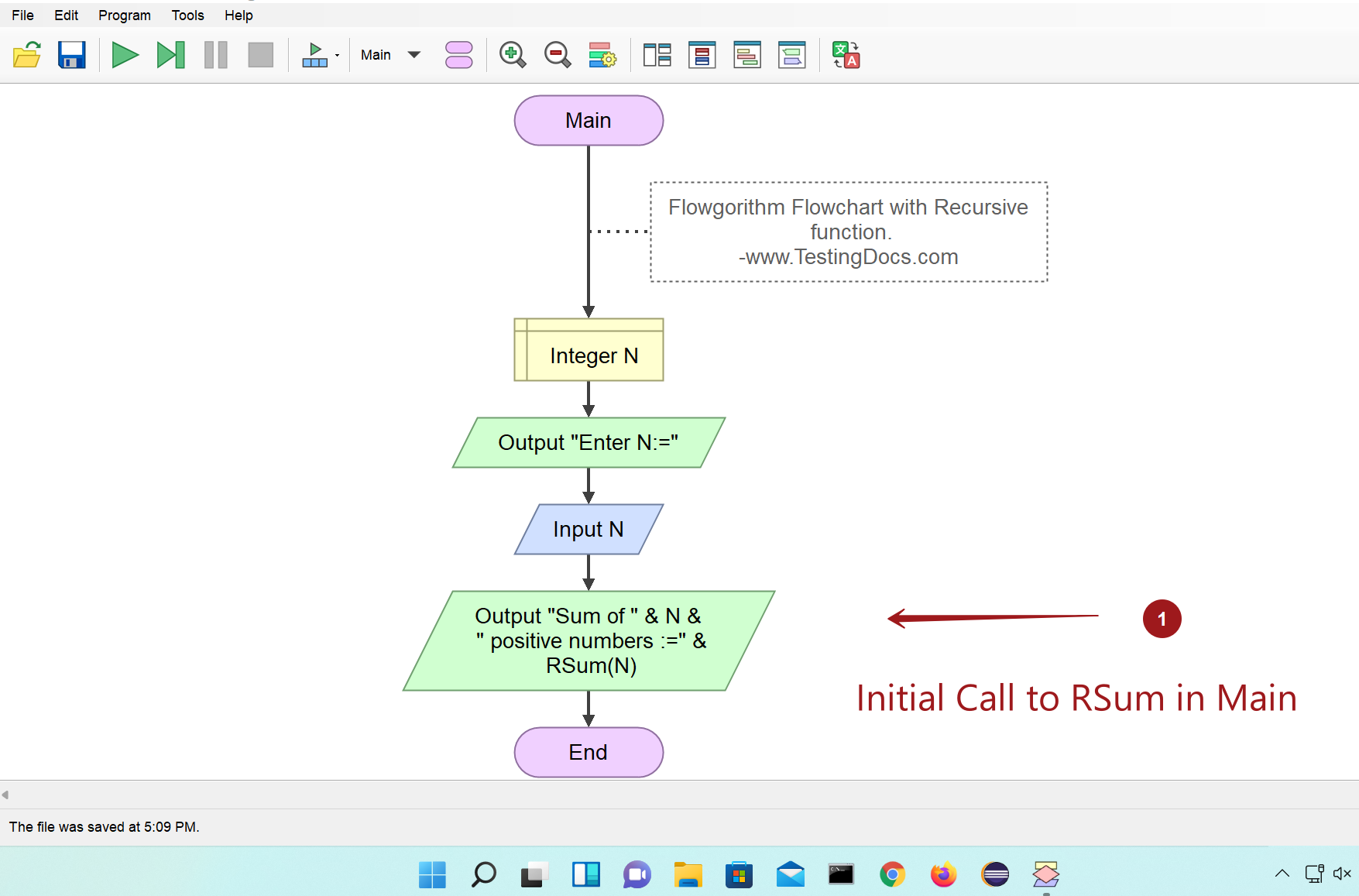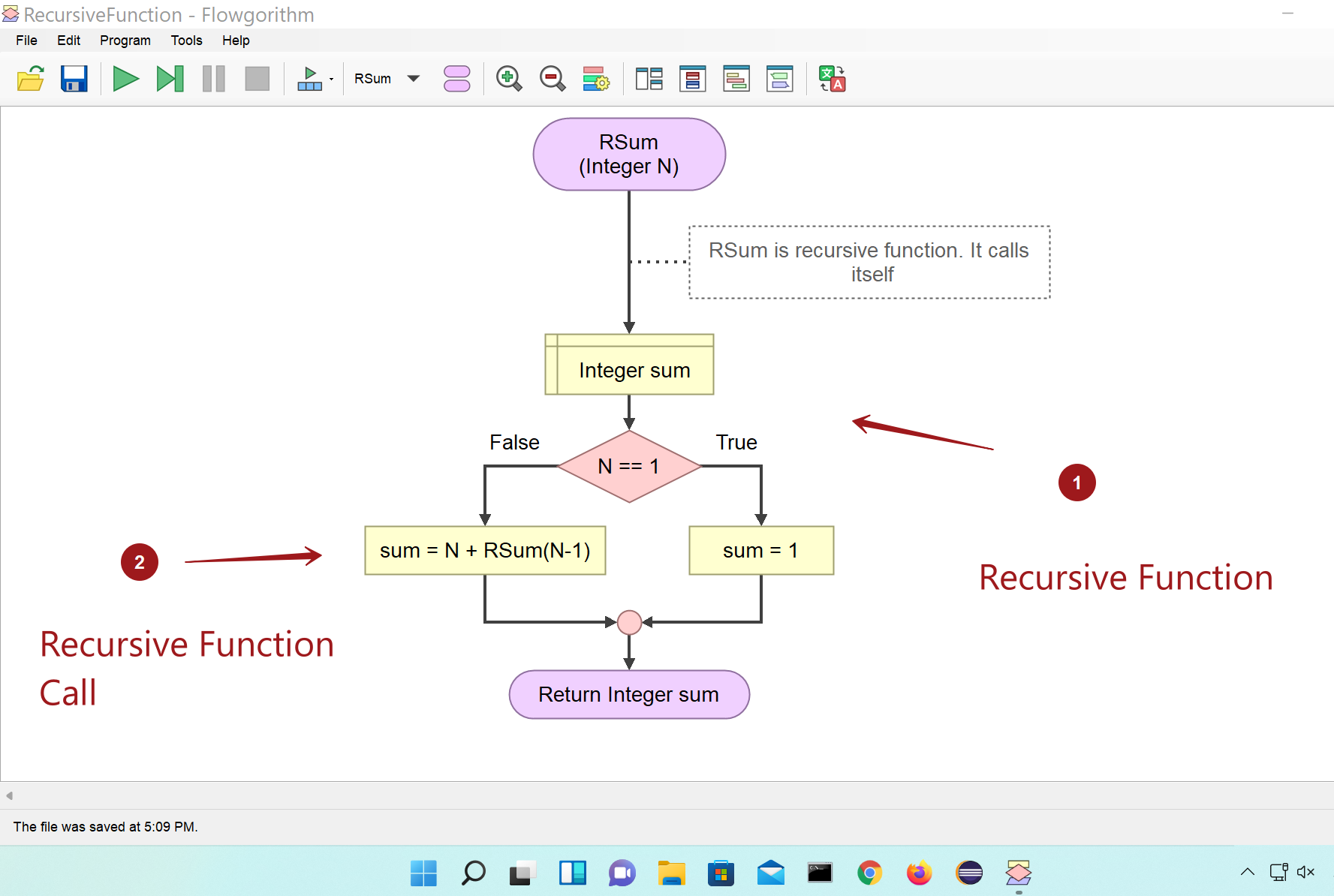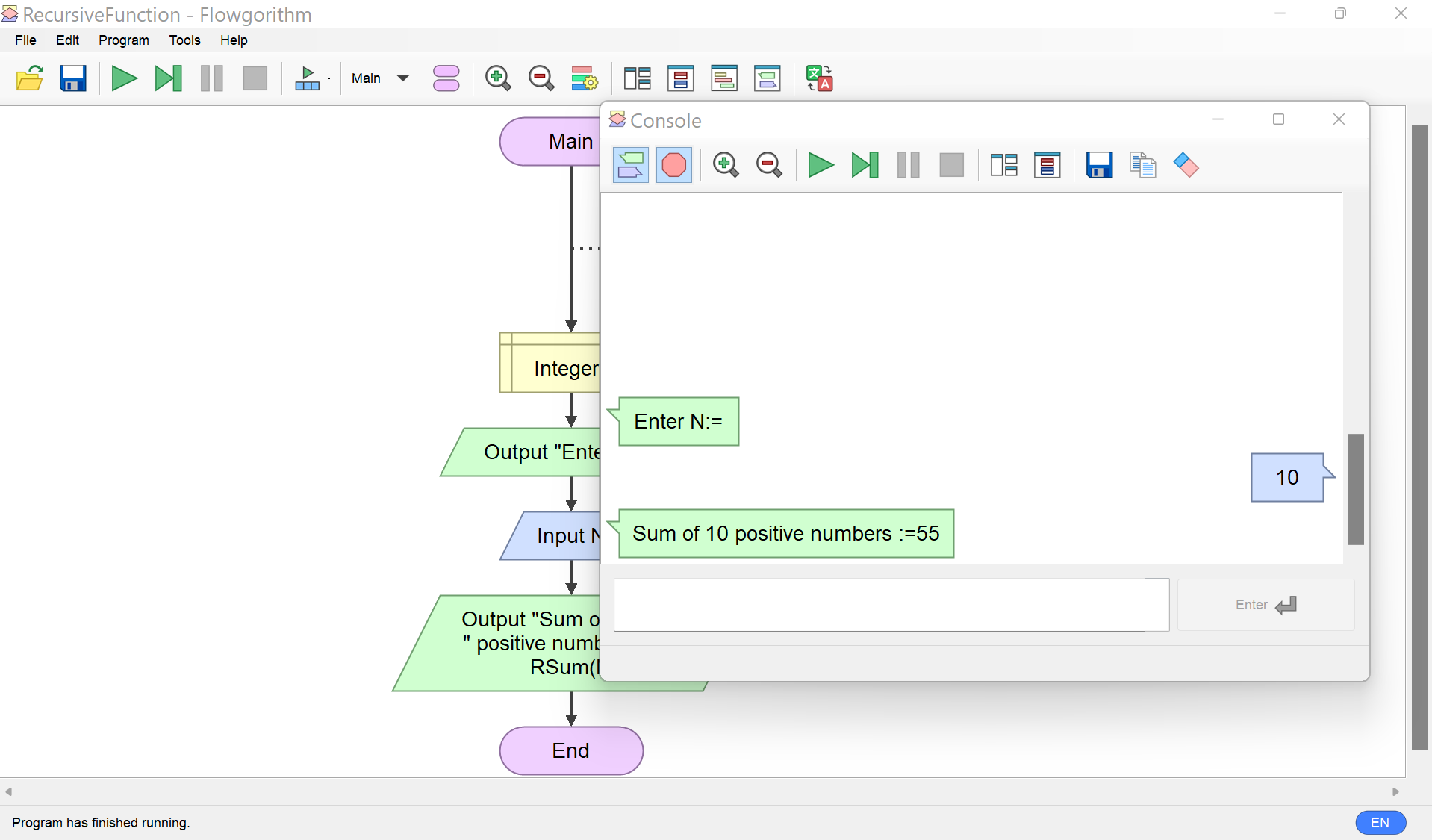# Overview

In this tutorial, we will understand Recursion using Flowgorithm flowchart. In the earlier example using Function, we have noticed that the Main flowchart calls the function. We will design a recursive function called RSum to compute the sum of n natural numbers.

## Recursive Function

A recursive function is a function that invokes itself. Recursion occurs when the function defines in the terms of itself. Flowgorithm supports recursion. We will use the Call statement within the function definition to call itself.

## Mathematical Notation

The Sum of n natural numbers can be denoted as the following Mathematical notation:

Alternatively, we can define the sum series as:

## Recursive calls

Let’s design a recursive function to compute the sum of N positive numbers called RSum. We need to define the base case and the recursive case.

Sum of n numbers in recursive format:

Base case: When n= 1 the sum is 1. This is the halting case. We should tell the function to stop the recursive calls.

Recursive case:

RSum = n + RSum(n-1)

This in turn changes in the next recursion:

RSum = n + (n-1) + RSum(n-2)

and so on until we reach the base case.

## Example Flowchart

The Main function prompts the user for the number N. The Main function invokes the recursive function with the parameter N. RSum is the recursive function.

Main Flowchart## Recursive function: RSum

The recursive function RSum calls itself to compute the sum of N natural numbers.

The function parameter is Integer N. The function returns the sum. We can notice that the function invokes itself.## Sample Output

Execute the Main flowchart and verify the output.If the flowchart input is N the flowchart output of the recursive function should be:

N*(N+1)/2

For example, for input 10 the output should be :

= 10*(10+1)/2

= (10*11)/2

= 55

That’s it. We have successfully created a flowchart with a recursive function using Flowgorithm. The common error in designing the recursive functions is Infinite recursion.

Infinite recursion: Infinite Recursion Example

## Flowgorithm Tutorials

Flowgorithm flowchart tutorials on this website:

https://www.testingdocs.com/flowgorithm-flowchart-tutorial/

Flowgorithm Website